Work, Energy, and Power - Lesson 2 - The Work-Energy Relationship

# Analysis of Situations in Which Mechanical Energy is Conserved

It has previously been mentioned that there is a relationship between work and mechanical energy change. Whenever work is done upon an object by an external force (or nonconservative force), there will be a change in the total mechanical energy of the object. If only internal forces are doing work (no work done by external forces), then there is no change in the total amount of mechanical energy. The total mechanical energy is said to be conserved. In this part of Lesson 2, we will further explore the quantitative relationship between work and mechanical energy in situations in which there are no external forces doing work.

The quantitative relationship between work and the two forms of mechanical energy is expressed by the following equation:

KEi + PEi + Wext = KEf + PEf

The equation illustrates that the total mechanical energy (KE + PE) of the object is changed as a result of work done by external forces. There are a host of other situations in which the only forces doing work are internal or conservative forces. In such situations, the total mechanical energy of the object is not changed. The external work term cancels from the above equation and mechanical energy is conserved. The previous equation is simplified to the following form:

KEi + PEi = KEf + PEf

In these situations, the sum of the kinetic and potential energy is everywhere the same. As the potential energy is increased due to the stretch/compression of a spring or an increase in its height above the earth, the kinetic energy is decreased due to the object slowing down. As the potential energy is decreased due to the return of a spring to its rest position or a decrease in height above the earth, the kinetic energy is increased due to the object speeding up. We would say that energy is transformed or changes its form from kinetic energy to potential energy (or vice versa); yet the total amount present is conserved - i.e., always the same.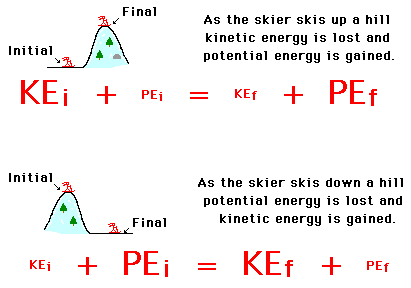### The Example of Pendulum Motion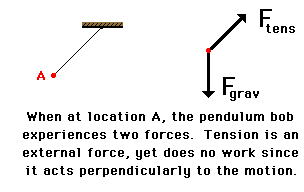The tendency of an object to conserve its mechanical energy is observed whenever external forces are not doing any overall work. If the influence of friction and air resistance can be ignored (or assumed to be negligible) and all other external forces are absent or merely not doing work, then the object is often said to conserve its energy. Consider a pendulum bob swinging to and fro on the end of a string. There are only two forces acting upon the pendulum bob. Gravity (an internal force) acts downward and the tensional force (an external force) pulls upwards towards the pivot point. The external force does not do work since at all times it is directed at a 90-degree angle to the motion. (Review a previous page to convince yourself that F•d•cosine angle = 0 J for the force of tension.)

As the pendulum bob swings to and fro, its height above the tabletop (and in turn its speed) is constantly changing. As the height decreases, potential energy is lost; and simultaneously the kinetic energy is gained. Yet at all times, the sum of the potential and kinetic energies of the bob remains constant. The total mechanical energy is 6 J. There is no loss or gain of mechanical energy, only a transformation from kinetic energy to potential energy (and vice versa). This is depicted in the diagram below.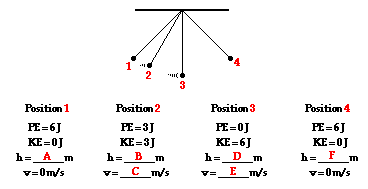As the 2.0-kg pendulum bob in the above diagram swings to and fro, its height and speed change. Use energy equations and the above data to determine the blanks in the above diagram. Click the button to view answers.

### Learning From Lab

A common Physics lab involves the analysis of a pendulum in its back and forth motion. The transformation and conservation of mechanical energy is the focus of the lab.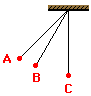A 0.200-kg (200 gram) pendulum is typically released from rest at location A. The bob passes through a photogate at location B and another photogate at location C. The speed of the pendulum bob can be determined from the width of the bob and the photogate times. The speed and mass can be used to determine the kinetic energy of the bob at each of the three locations. The heights of the bob above the tabletop at each of the three locations can be measured and used to determine the potential energy of the bob. The data should reflect that the mechanical energy changes its form as the bob passes from location A to B to C. Yet the total mechanical energy should remain relativity constant. Sample data for such a lab are shown below.

 Loc'n Height Speed PE KE TME A 0.400 m 0 m/s 0.784 J 0 J 0.784 J B 0.248 m 1.70 m/s 0.486 J 0.289 J 0.775 J C 0.096 m 2.47 m/s 0.188 J 0.610 J 0.798 J

The sample data show that the pendulum bob loses potential energy as it swings from the more elevated location at A to the lower location at B and at C. As this loss of potential energy occurs, the pendulum bob gains kinetic energy. Yet the total mechanical energy remains approximately 0.785 Joules. We would say that total mechanical energy is conserved as the potential energy is transformed into kinetic energy.### The Example of a Roller Coaster

A roller coaster operates on this same principle of energy transformation. Work is initially done on a roller coaster car to lift to its initial summit. Once lifted to the top of the summit, the roller coaster car has a large quantity of potential energy and virtually no kinetic energy (the car is almost at rest). If it can be assumed that no external forces are doing work upon the car as it travels from the initial summit to the end of the track (where finally an external braking system is employed), then the total mechanical energy of the roller coaster car is conserved. As the car descends hills and loops, its potential energy is transformed into kinetic energy as the car speeds up. As the car climbs up hills and loops, its kinetic energy is transformed into potential energy as the car slows down. Yet in the absence of external forces doing work, the total mechanical energy of the car is conserved.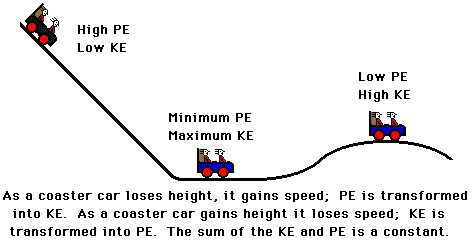Conservation of energy on a roller coaster ride means that the total amount of mechanical energy is the same at every location along the track. The amount of kinetic energy and the amount of potential energy is constantly changing. Yet the sum of the kinetic and potential energies is everywhere the same. This is illustrated in the diagram below. The total mechanical energy of the roller coaster car is a constant value of 40 000 Joules.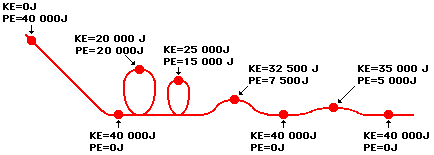### The Example of a Ski Jumper

The motion of a ski jumper is also governed by the transformation of energy. As a ski jumper glides down the hill towards the jump ramp and off the jump ramp towards the ground, potential energy is transformed into kinetic energy. If it can be assumed that no external forces are doing work upon the ski jumper as it travels from the top of the hill to the completion of the jump, then the total mechanical energy of the ski jumper is conserved. Consider Lee Ben Fardest (esteemed American ski jumper). He starts at rest on top of a 100-meter hill, skis down the 45-degree incline and makes a world record setting jump. Assuming that friction and air resistance have a negligible effect upon Lee's motion and assuming that Lee never uses his poles for propulsion, his total mechanical energy would never change.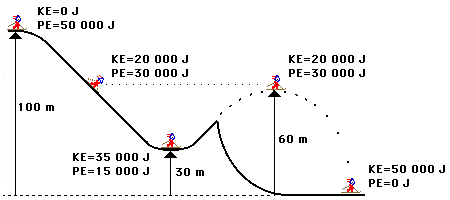Of course it should be noted that the original assumption that was made for both the roller coaster car and the ski jumper is that there were no external forces doing work. In actuality, there are external forces doing work. Both the roller coaster car and the ski jumper experience the force of friction and the force of air resistance during the course of their motion. Friction and air resistance are both external forces and would do work upon the moving object. In fact, the presence of friction and air resistance would do negative work and cause the total mechanical energy to decrease during the course of the motion. While the assumption that mechanical energy is conserved is an invalid assumption, it is a useful approximation that assists in the analysis of an otherwise complex motion.

Click to try several practice/application problems.

### We Would Like to Suggest ...Sometimes it isn't enough to just read about it. You have to interact with it! And that's exactly what you do when you use one of The Physics Classroom's Interactives. We would like to suggest that you combine the reading of this page with the use of our Roller Coaster Model Interactive, our Mass on a Spring Interactive, and/or our Chart That Motion Interactive. These three Interactives can be found in the Physics Interactive section of our website and provide an interactive opportunity to explore the work-energy relationship.

Next Section:

Follow Us